# Basic Geometry : How to find the angle of clock hands

## Example Questions

← Previous 1

### Example Question #1 : How To Find The Angle Of Clock Hands

What is the angle formed by the minute hand and the hour hand at 4:45?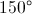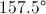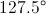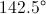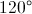Explanation:

The angle measure between any two consecutive numbers on a clock is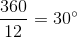.

Call the "12" point on the clock the zero-degree point.

At 4:45, the minute hand is at the "9" - that is, at the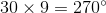mark. The hour hand is three-fourths of the way from the "4" to the "5; that is,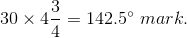Therefore, the angle between the hands is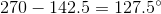, the desired measure.

### Example Question #1 : How To Find The Angle Of Clock Hands

What is the angle between the hour hand and the minute hand at 4:40?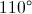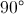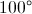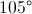Explanation:

At 4:40, the minute hand is on the 8, and the hour hand is two-thirds of the way from the 4 to the 5. That is, the hands are three and one-third number positions apart. Each number position is thirty degrees around the clock, so the hands form an angle of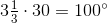.

### Example Question #1 : How To Find The Angle Of Clock Hands

It is 4 o’clock.  What is the measure of the angle formed between the hour hand and the minute hand?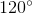Explanation:

At four o’clock the minute hand is on the 12 and the hour hand is on the 4.  The angle formed is 4/12 of the total number of degrees in a circle, 360.

4/12 * 360 = 120 degrees

### Example Question #1 : How To Find The Angle Of Clock Hands

The hour hand on a clockface points to the, and the minute hand points to the.  How many degrees is the angle between the minute and hour hands?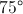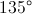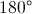Explanation:

There are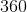degrees in one complete revolution of a circle. There areminutes in one hour.

Create a fraction out of these two quantities to use later as a conversion rate: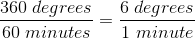Between theandthere areminutes, so multiply this by the conversion rate to solve for the number of degrees: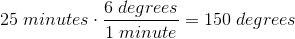### Example Question #3 : How To Find The Angle Of Clock Hands

What is the measure of the smaller angle formed by the hands of an analog watch if the hour hand is on the 10 and the minute hand is on the 2?

120°

90°

56°

30°

45°

120°

Explanation:

A analog clock is divided up into 12 sectors, based on the numbers 1–12. One sector represents 30 degrees (360/12 = 30). If the hour hand is directly on the 10, and the minute hand is on the 2, that means there are 4 sectors of 30 degrees between then, thus they are 120 degrees apart (30 * 4 = 120).

### Example Question #4 : How To Find The Angle Of Clock Hands

Thomas is trying to determine the angle between the hands of his clock. Right now it reads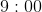pm, what angle do the clock hands make?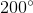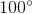Explanation:

You can think of a clock in two ways:

1. Out of 12 hours, or

2. In terms of a circle with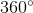If you try to solve it in terms of #1:

Goal: Find the angle measurement between the hour and the minute hands. We only want to find the degrees between the hours of 9 and 12

So we are looking at 3 hours out of the 12 total hours on a clock.

As a fraction: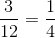So that means that the clock hands are making an angle that is 1/4 of the clock (which is a circle). So knowing that a circle hasin it,

1/4 of a circle is.

____________________________________________________________

2. If you think of the clock as a circle first you can determine the angle that the clock hands create very quickly.

Since there arein a circle, every hour that passes is a movement of. So knowing that, the clock will be moving 3 hours: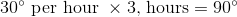### Example Question #2 : How To Find The Distance Between Clock Hands

What is the measure of the larger angle formed by the hands of a clock at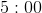?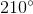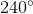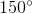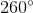Explanation:

Like any circle, a clock contains a total of. Because the clock face is divided intoequal parts, we can find the number of degrees between each number by doing. At 5:00 the hour hand will be at 5 and the minute hand will be at 12. Using what we just figured out, we can see that there is an angle ofbetween the two hands. We are looking for the larger angle, however, so we must now do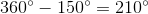### Example Question #1 : How To Find The Angle Of Clock Hands

What is the measure, in degrees, of the acute angle formed by the hands of a 12-hour clock that reads exactly 3:10?

35°

65°

72°

55°

60°

35°

Explanation:

The entire clock measures 360°. As the clock is divided into 12 sections, the distance between each number is equivalent to 30° (360/12). The distance between the 2 and the 3 on the clock is 30°.  One has to account, however, for the 10 minutes that have passed. 10 minutes is 1/6 of an hour so the hour hand has also moved 1/6 of the distance between the 3 and the 4, which adds 5° (1/6 of 30°). The total measure of the angle, therefore, is 35°.

### Example Question #5 : How To Find The Angle Of Clock Hands

Find the angle in degrees between clock hands at 3:30.Explanation:

On first glance, this problem may seem simple in that the angle between the 3 and 6 on a clock is one quarter of a circle or 90 degrees. However, you must take into account that in the half hour that has passed from 3:00 to 3:30, the hour hand has moved half the distance between the 3 and 4. To find the degrees, simply divide the total number of degrees in a circle by 12 to find the degrees between each consecutive number, and then multiply that number by 2.5 because you have half the distance to the 4, and the the full distance to the 5 and the full distance to the 6. Thus,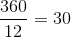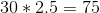### Example Question #6 : How To Find The Angle Of Clock Hands

A clock shows that the time is 9:00am. What is the angle between the minute and the hour hands?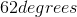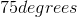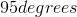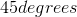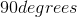Explanation:

By dividing the clock into pieces, we can determine that the angle between the two hands is.

Within a clock, just like any circle, there are 360 total degrees

Within an clock, there are 60 total minutes.

Each minute that passes, the minute hand advances 6 degrees. The hour hand advances .5 degrees. But since it is 9am on the dot, we will just be using the minute hand to count the degrees.

Since the minute hand is covering a total of 15 minutes in between it and the hour hand, we can do the math: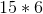=← Previous 1